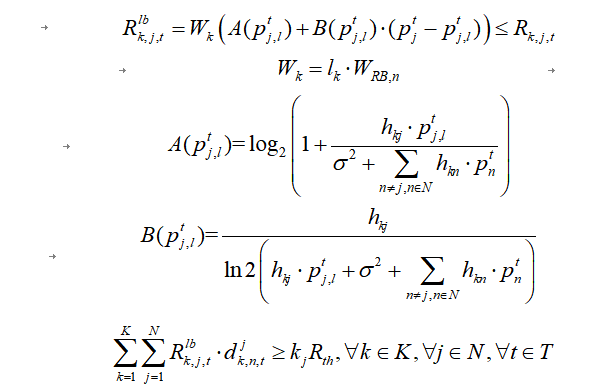# How to resolve this code error?（ Invalid constraint: {convex} >= {real constant}）

My Convex Constraint Formula：My convex constraint formula is written as code:
sum_except_i = sum(H_N_Kb_all(n,.* (l_N_k .*Pl(n))) - H_N_Kb_all(n, i) * Pl(n) * l_N_k(i);
A_Pl = (log(1 + (H_N_Kb_all(n, i) * Pl(n) * l_N_k(i)) )/ log(2) )./ (N_noise + sum_except_i);
B_pl = (H_N_Kb_all(n, i) * l_N_k(i)) ./ (log(2) * (H_N_Kb_all(n, i) * Pl(n) * l_N_k(i) + N_noise + sum_except_i));
for n = 1:N_bs
sum_Rlb_n_i(i) = 0;
for i = 1:k_all
Rlb_n_i = l_N_k(i)*W_bs_RB(n) * (A_Pl + B_pl * abs(P(n) - Pl(n)));
sum_Rlb_n_i(i) = sum_Rlb_n_i(i) + A_N_K_up_low(n, i) * Rlb_n_i;
end
sum_Rlb_n_i(i) >= R_sum_min ;
end
Among them, (pj, l) is a variable, while the others are constants.
The error:
Disciplined convex programming error:
Invalid constraint: {convex} >= {real constant}

error >= (line 21)
b = newcnstr( evalin( ‘caller’, ‘cvx_problem’, ‘[]’ ), x, y, ‘>=’ );

error main_problem (line 337)
sum_Rlb_n_i(i) >= R_sum_min ;

You haven’t show a complete program, with all variable declarations. Nor do I know what values the input data has Nor how your program supposedly corresponds to the problem definition shown in the image…

Nevertheless, based on the error message, it appears that `Rlb_n_i(i)` and hence `sum_Rlb_n_i(i) ` are convex due to `abs(P(n) - Pl(n)))`. The error message then occurs because `convex >= constant` is a non-convex constraint. Note that `concave >= constant` and ``convex <= constant` are convex constraints.

Whether the non-convex constraint in your code is really supposed to be the constraint is for you to determine, since I don’t understand what your problem actually is.given the hodgepodge of symbols with no clear definition as to which are optimization variables, and what any unstated relations may be.

1 Like

The overall code for my question is as follows：
Pl = p_N_t;
l = 0;
sum_except_i = sum(H_N_Kb_all(n,.* (l_N_k .*Pl(n))) - H_N_Kb_all(n, i) * Pl(n) * l_N_k(i);
A_Pl = (log(1 + (H_N_Kb_all(n, i) * Pl(n) * l_N_k(i)) )/ log(2) )./ (N_noise + sum_except_i);
B_pl = (H_N_Kb_all(n, i) * l_N_k(i)) ./ (log(2) * (H_N_Kb_all(n, i) * Pl(n) * l_N_k(i) + N_noise + sum_except_i));
cvx_clear
cvx_quiet(true)
cvx_begin
variables P(N_bs, 1)
expression sum_Rlb_n_i(k_all)
minimize (sum(-log(P) / log(10)))
subject to:
for n = 1:N_bs
sum_Rlb_n_i(i) = 0;
for i = 1:k_all
Rlb_n_i = l_N_k(i)*W_bs_RB(n) * (A_Pl + B_pl * abs(P(n) - Pl(n)));
sum_Rlb_n_i(i) = sum_Rlb_n_i(i) + A_N_K_up_low(n, i) * Rlb_n_i;
end
sum_Rlb_n_i(i) >= R_sum_min ;
end
In these codes:
The dimension of matrix (HN_Kb_all) is (N_bs, k_all)；The dimension of matrix (l_N_k) is (1, k_all)；The dimension of matrix ( p_N_t) is (N_bs, 1).
The definitions of some constants in the code are:
N_bs=4;k_all = 100;K_b=25;
Based on your previous reply, I need to set the constraint (sum_Rlb_n_i (i)>=R_ Sum_ Rewrite as “concave>=constant” or “convex<=constant” constraint. So I can change this constraint to a convex constraint?
concave>=constant” or “convex<=constant” would be accepted by CVX, but they are NOT equivalent to your code. I.e., `sum_Rlb_n_i(i) <= R_sum_min` would be accepted by CVX, but it is a different model. You have to determine what model is correct for the phenomenon you are modeling.